Tamilnadu State Board New Syllabus Samacheer Kalvi 11th Maths Guide Pdf Chapter 2 Basic Algebra Ex 2.12 Text Book Back Questions and Answers, Notes.

## Tamilnadu Samacheer Kalvi 11th Maths Solutions Chapter 2 Basic Algebra Ex 2.12

Question 1.
Let b > 0 and b ≠ 1. Express y = bx in logarithmic form. Also, state the domain and range of the logarithmic function.
Given y = bx ⇒ logby = x, x ∈ R with range (0, ∞) (-∞, ∞)Question 2.
Compute log9 27 – log27 9
log927 – log279 = log9 33 – log27 32
= 3 log9 3 – 2 log27 3 —— (1)
By change of base rule [logb a = $$\frac{1}{\log _{a} b}$$]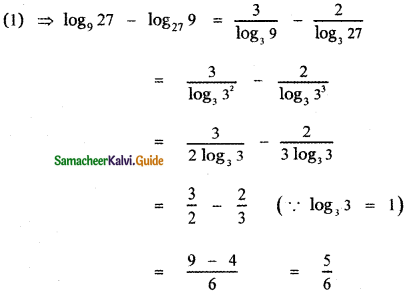Question 3.
Solve logax + log4x + log2x = 11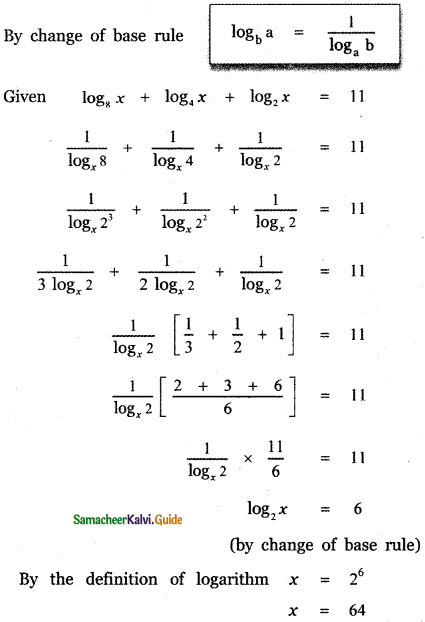Question 4.
Solve log 4 2 8x = 2 log28
Given log 4 28x = 2 log28
log 4 28x = 2log223
log 4 28x = 23 log22
log 4 28x = 23 = 8
28x = 48
(22)4x = 48
⇒ (4)4x = 48
⇒ 4x = 8
⇒ x = $$\frac { 8 }{ 4 }$$ = 2

Question 5.
If a2 + b2 = 7ab, show that
log $$\left(\frac{a+b}{3}\right)$$ = $$\frac{1}{2}$$ (log a + log b)
Given
a2 + b2 = 7ab
Adding both sides 2ab we get
a2 + b2 + 2ab = 7ab + 2ab
(a + b)2 = 9ab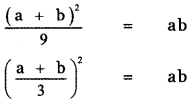Taking square root on both sides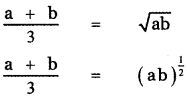Taking logarithm on both sides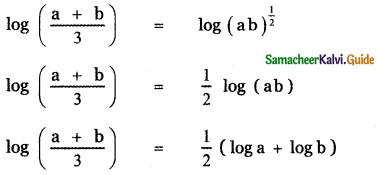Question 6.
Prove that log $$\frac{\mathbf{a}^{2}}{\mathbf{b c}}$$ + log $$\frac{\mathbf{b}^{2}}{\mathbf{c a}}$$ + log $$\frac{c^{2}}{a b}$$ = 0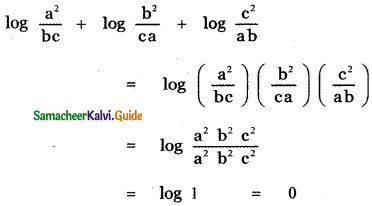Question 7.
Prove that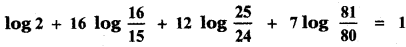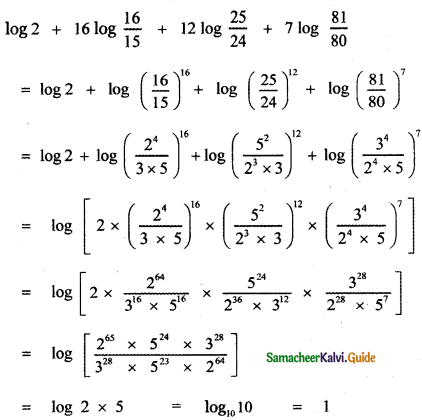Question 8.
Prove that loga2 a + logb2 b + logc2 c = $$\frac{1}{8}$$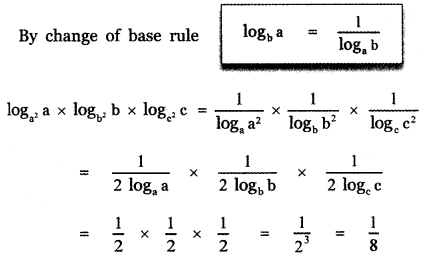Question 9.
Prove log a + log a2 + log a3 + ……… + log an = $$\frac{n(n+1)}{2}$$ log a
log a + log a2 + log a3 + ……… + log an
= log a + 2 log a + 3 log a + ………….. + n log a
= log a (1 + 2 + 3 + ………….. + n)
= log a × $$\frac{n(n+1)}{2}$$
= $$\frac{n(n+1)}{2}$$ log a

Question 10.
If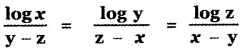, then prove that xyz = 1
Let $$\frac{\log x}{y-z}$$ = k
log x = k(y – z)
log x = ky – kz ——— (1)
Similarly log y = k(z – x) = kz – kx ——(2)
log z = k(x – y) = kx – ky ——- (3)
Adding (1), (2) and (3)
log x + log y + log z = ky – kz + kz – kx + kx – ky
log (xyz) = 0 = log 1
xyz = 1Question 11.
Solve log2x – 3 log1/2x = 6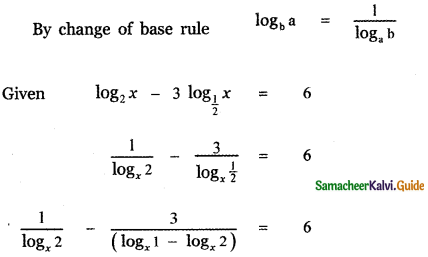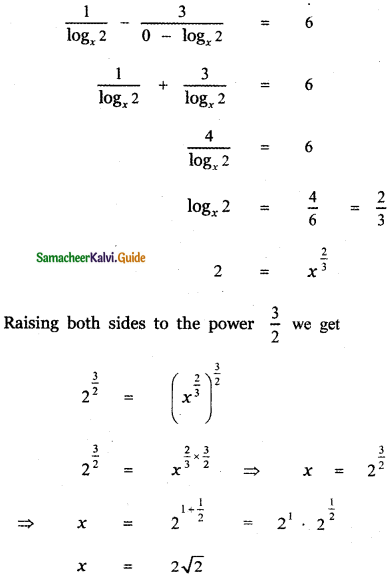Question 12.
Solve log5 – x (x2 – 6x + 65) = 2